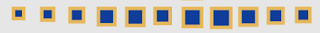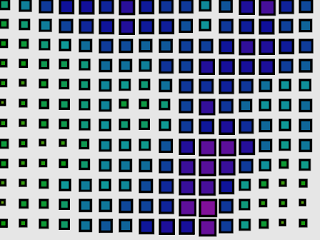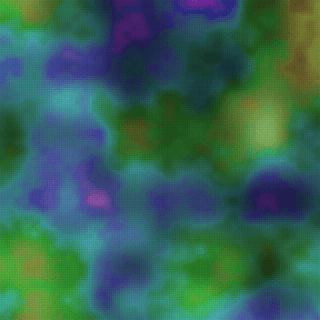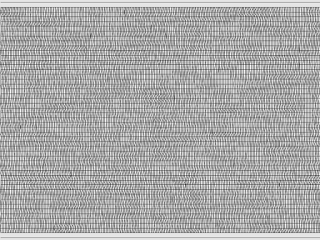# Come on! Feel the noise! Part 1

The 'noise' is a very useful feature in creative coding. But I heard many of them say 'I don't know how to use it' 'It's a little bit hard to understand' etc.

This is my article for beginners who wants to understand 'noise()' and want to know how to use it. Let me show you the features of 'noise()' and the example code using 'noise()'.

Let's enjoy with me to do creative coding using 'noise()'!

Note.
I only explain the 'noise()' function in Processing and p5.js.
The example codes in this article are written in Processing. And I linked to the OpenProcessing for codes of p5.js. And I will share these codes under creative commons CC0 (except example works). Please feel free to use these codes.

## The random value that changes smoothly.

'noise()' is 'The random value that changes smoothly' in one sentence.

I'm sure that you used the 'random()' function before.
For example, you can draw a graph with a code like this.

`    float y = random(height);`
If you used 'noise()', the graph will look like this.How smooth is this! 😲

If you painted with random colors.Ummm... disordering look.
Then, with noise.I did not add a color gradation function. I just used 'noise()' instead of 'random()'.

Just as above, you can get smooth random values from 'noise()'.
Let's see the code that draws the graph above.
``````
/**
* Come on! Feel the noise! Part 1
* random/noise graph
*
* @author @deconbatch
* @version 0.1
* Processing 3.5.3
* 2020.10.31
*/

void setup() {
size(640, 480);
colorMode(HSB, 360.0, 100.0, 100.0, 100.0);
noLoop();

background(0.0, 0.0, 90.0, 100.0);
noFill();
stroke(0.0, 0.0, 0.0, 100.0);
strokeWeight(2.0);
}

void draw() {
int div = 50;
beginShape();
for (int i = 0; i < div; i++) {
float x = i * width / div;
//    float y = random(height);
float y = noise(i * 0.05) * height;
vertex(x, y);
ellipse(x, y, 10.0, 10.0);
}
endShape();
}
```
```

The key of this code is here.

`    //    float y = random(height);    float y = noise(i * 0.05) * height;`

The commented random(height) returns from 0 to before 'height' value as you know.
And noise(i * 0.05) returns the random value between 0 to 1.

### Wait! Then what is 'i * 0.05'?

I know, I know. 😌
'noise()' returns the value between 0 to 1 even for any parameter value.
Let me write a code like this.

`    float y = noise(height);`

The result is like this.Oops!😣 'noise()' returns the value between 0 to 1. So 0 < y < 1. Let's multiply by 100. Then 0 < y < 100.
`    float y = noise(height) * 100.0;`

Hmmm. It looks like a straight line...? 🤔
You are right!

'noise()' has the feature

1.'noise()' returns the value between 0 to 1 even for any parameter value.

and

2.'noise()' returns the same value for the same parameter value.

Then, what happens with this code that changes the parameter value with 'i'.

`    float y = noise(i * 0.05) * 100.0;`
Yes! The line curves with the 'noise(i * 0.05)'.

Let's run the same code again.

Oh!? It drew a different result! 😳
I said 'noise() returns the same value for the same parameter value' before. It is only in the same execution of Processing/p5.js

Even if you give the same parameters, it returns different results for each execution.

### How smooth it can be?

You can see this curve changes smoothly from left to right. In the code, the value of 'y' changes smoothly when the value of 'i' increases.

It means

3.The return value of 'noise()' changes smoothly when the parameter value change.

So, how smooth it is?
It depends on the change rate of a parameter. For example, the code
`    float y = noise(i * 0.05) * 100.0;`
Let's multiply by 1/10.
`    float y = noise(i * 0.005) * 100.0;`

Wow! It's toooooo smoooooth!

Then, multiply by 10.

`    float y = noise(i * 0.5) * 100.0;`

Too rough! It just looks like a 'random()'!

4.Smoothness depends on the change rate of a parameter.

As above, the change rate of a parameter is the key to the resulting smoothness.
I recommend to try from 0.05 to 0.1 rate of a parameter first and tune by the result.

## The same result with the same parameter absolute value.

I said 'noise() returns the same value for the same parameter value'. It returns the same value even if you multiply minus and the parameter.

So, actually,

2.'noise()' returns the same value for the same parameter ABSOLUTE value.

You can draw a symmetrical shape with this feature.

``````
/**
* Come on! Feel the noise! Part 1
* With the same parameter absolute value.
*
* @author @deconbatch
* @version 0.1
* Processing 3.5.3
* 2020.10.31
*/

void setup() {
size(640, 480);
colorMode(HSB, 360.0, 100.0, 100.0, 100.0);
noLoop();

background(0.0, 0.0, 90.0, 100.0);
stroke(0.0, 0.0, 0.0, 100.0);
strokeWeight(1.0);
}

void draw() {
int div = 50;
float w = width * 0.5 / div;
float h = height * 0.5 / div;

translate(width * 0.5, height * 0.5);
for (int i = -div; i < div; i++) {
for (int j = -div; j < div; j++) {
float x = i * w;
float y = j * h;
fill(noise(i * j * 0.01) * 360.0, 80.0, 90.0, 100.0);
rect(x, y, w, h);
}
}
}

```
```

Link to the p5.js code on OpenProcessing.

Putting some flavor.
Summary.
1. 'noise()' returns the value between 0 to 1 even for any parameter value.
2. 'noise()' returns the same value for the same parameter absolute value.
3. The return value of 'noise()' changes smoothly when the parameter value change.
4. Smoothness depends on the change rate of a parameter.

## The dimension of the parameter.

It is how to use rather than a feature.
As you see in the reference of Processing/p5.js, 'noise()' takes one, two, and three parameters.

We call the 'noise()' with one parameter as '1D noise'. Two as '2D noise', three as '3D noise'.

// one, two, and three parameters.
noise(x)        // 1D noise
noise(x, y)     // 2D noise
noise(x, y, z)  // 3D noise

We have used 1D noise so far.
The feature of 'noise()' described before is the same in 1D, 2D, and 3D noise.

Let's write a code like this with 2D noise.
``````
/**
* Come on! Feel the noise! Part 1
* Let's observe 2D noise in the line graph.
*
* @author @deconbatch
* @version 0.1
* Processing 3.5.3
* 2020.10.31
*/

void setup() {
size(640, 320);
colorMode(HSB, 360.0, 100.0, 100.0, 100.0);
noLoop();

background(0.0, 0.0, 90.0, 100.0);
noFill();
stroke(0.0, 0.0, 0.0, 100.0);
strokeWeight(2.0);
}

void draw() {
int divX = 50;
int divY = 5;
float w = width  / divX;
float h = height / divY;

for (int j = 0; j < divY; j++) {
beginShape();
for (int i = 0; i < divX; i++) {
float x = i * w;
float y = noise(i, j) * 100 + j * h;
vertex(x, y);
}
endShape();
}
}

```
```

Here is the 2D noise.

`      float y = noise(i, j) * 100 + j * h;`
The result is like this.

It is a little bit rough. Let's reduce the change rate value of the x-axis.

`      float y = noise(i * 0.1, j) * 100 + j * h;`
It made smooth the curve change in the x-axis direction.
Then, what happens when we reduce the change rate value of the y-axis?
`      float y = noise(i, j * 0.1) * 100 + j * h;`

You can see these lines are similar in shape to each other.
If we set the change rate value of the y-axis to zero (no change).

`      float y = noise(i, j * 0.0) * 100 + j * h;`

Now, all lines are the same shape. It means 'noise(i, no change parameter)' run as 1D noise.

## Fun time! Let's make some images with 2D noise.

Let's make some images with 2D noise.

``````
/**
* Come on! Feel the noise! Part 1
* THIS IS THE 2D NOISE!
*
* @author @deconbatch
* @version 0.1
* Processing 3.5.3
* 2020.10.31
*/

void setup() {
size(640, 480);
colorMode(HSB, 360.0, 100.0, 100.0, 100.0);
noLoop();

background(0.0, 0.0, 90.0, 100.0);
fill(220.0, 90.0, 60.0, 100.0);
stroke(40.0, 60.0, 90.0, 100.0);
strokeWeight(5.0);
}

void draw() {
int divX = 16;
int divY = 12;
float w = width  / divX;
float h = height / divY;

for (int i = 0; i < divX; i++) {
float x = i * w;
for (int j = 0; j < divY; j++) {
float y = j * h;
float s = noise(x * 0.005, y * 0.005);
rect(x, y, s * w, s * h);
}
}
}

```
```

I applicate the 2D noise to the rectangle size. You can see these size change smoothly from left to right and top to bottom.

In this 2D noise,

`noise(x * 0.005, y * 0.005)`

If 'x' value was the same, the sizes in the y-axis change smoothly.And 'y' value was the same, the sizes in the x-axis change smoothly.So, every rectangle's neighbors have a little bit of difference.As above, when we treat 2D noise's parameter as x-axis and y-axis, we get smoothly changing random value even if we moves vertical, horizontal, and slantingly.
This is the 2D noise!

We can applicate to the colors.More further.And you may have seen this before.I call it 'leg hair'. 🦵
If you made it smaller, it becomes 'leg hair map'. 🤣And you can make an interesting application of this called 'Noise field' of 'Vector field'.
Here are my example creative coding works of the Vector Field.

If it's your first time to use 'noise()', it's good to make some graph with 1D noise.These are the graphs with many vertical lines with 1D noise.You can enjoy tunning the change rate value of the parameter. It will show us different faces with the same code as above.

### Some example works.

This code does not display any images on the screen but generates image files in frames directory.
Sorry, it's Processing code only.

``````
/**
* Behind Closed Doors.
* It creates still images with noise().
*
* @author @deconbatch
* @version 0.1
* Processing 3.5.3
* 2020.11.23
*/

void setup() {

size(600, 360);
colorMode(HSB, 360.0, 100.0, 100.0, 100.0);
noLoop();

}

void draw() {

int   frmMax  = 3;
int   len     = max(width, height);
float pA      = random(0.01, 0.15);
float pB      = random(0.01, 0.15);
float baseHue = random(360);

for (int frmCnt = 0; frmCnt < frmMax; frmCnt++) {
baseHue += 120.0;
pA += 0.08;
pB += 0.04;
pA %= 0.15;
pB %= 0.15;

pushMatrix();
translate(len * 0.5, len * 0.5);
rotate(HALF_PI * floor(random(4.0)));
translate(-len * 0.5, -len * 0.5);
background(0.0, 0.0, 90.0, 100.0);
noStroke();
for (int x = 0; x < len; x++) {
for (int y = 0; y < len; y++) {
float distLT = dist(x, y, width * 0.2, height * 0.2);
float distRB = dist(x, y, width * 0.8, height * 0.8);
float nHue = noise(x * 0.008, y * 0.008, customNoise(distLT * pA, distRB * pB) * 2.0);
float nBri = noise(x * 0.008, y * 0.008, customNoise(distLT * pA, distRB * pB) * 4.0);
fill((baseHue + nHue * 240.0) % 360.0, 40.0 + nHue * 40.0, 10.0 + nBri * 80.0, 100.0);
rect(x, y, 1.0, 1.0);
}
}
popMatrix();

casing();
saveFrame("frames/" + String.format("%04d", frmCnt + 1) + ".png");
}

exit();

}

/**
* customNoise : custom noise function
*/
float customNoise(float _a, float _b) {
return sin(_a) - cos(_b);
}

/**
* casing : draw fancy casing
*/
public void casing() {
fill(0.0, 0.0, 0.0, 0.0);
strokeWeight(50.0);
stroke(0.0, 0.0, 30.0, 100.0);
rect(0.0, 0.0, width, height);
strokeWeight(42.0);
stroke(0.0, 0.0, 100.0, 100.0);
rect(0.0, 0.0, width, height);
}

/*

This program is free software: you can redistribute it and/or modify
the Free Software Foundation; either version 3 of the License, or
(at your option) any later version.

This program is distributed in the hope that it will be useful,
but WITHOUT ANY WARRANTY; without even the implied warranty of
MERCHANTABILITY or FITNESS FOR A PARTICULAR PURPOSE.  See the
GNU General Public License for more details.

You should have received a copy of the GNU General Public License
along with this program.  If not, see <http://www.gnu.org/licenses/>
*/
```
```

And these tweet of mine are various example works with 'noise()'. Please use it as reference.